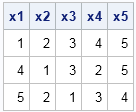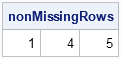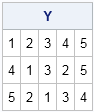SAS procedures usually handle missing values automatically. Univariate procedures such as PROC MEANS automatically delete missing values when computing basic descriptive statistics. Many multivariate procedures such as PROC REG delete an entire observation if any variable in the analysis has a missing value. This is called listwise deletion or using complete cases. Some procedures such as PROC FREQ and PROC CORR have options that control the way that missing values are handled in the statistical analysis.

Because SAS procedures internally handle which observations are kept or deleted, there is not usually a need for SAS programmers to create a data set that contains all of the complete cases. In contrast, SAS/IML programmers often have to worry about missing values. When implementing a multivariate algorithm, the first step is often to delete rows of the data matrix that contain missing values. This article shows how to perform listwise deletion by using the DATA step and PROC IML.

The case of missing values in numerical data is the most important case, so this article uses the following data set. The first, fourth, and fifth observations represent complete cases.

```data A; input x1-x5; datalines; 1 2 3 4 5 2 2 . 4 5 3 3 3 3 . 4 1 3 2 5 5 2 1 3 4 6 . . . 7 . 8 9 0 . . . . . . 9 8 7 . . ;```

### Output complete cases by using the DATA step

If the variables are all numeric, you can use the NMISS function to determine which observations have missing values. You can use the subsetting IF statement to output only those observations that do not contain missing values, as follows:

```data CompleteCases; set A; if nmiss(of _NUMERIC_)=0; /* output complete cases for all numeric vars */ run;   proc print noobs; run;```In the previous example, all numeric variables are used to determine the complete cases. You can also specify a list of variables to the NMISS function, as follows:

` if nmiss(x1, x2, x5)=0; /* specify list of numeric vars */`

For character variables, you can use the CMISS function and the _CHARACTER_ keyword. In fact, the CMISS function also counts numeric variables, so it enables you to handle numeric and character variables with a single call. The following DATA step outputs the complete cases for all variables in the Sashelp.Heart data set:

```data CompleteCases; set Sashelp.Heart; if cmiss(of _ALL_)=0; /* complete cases for all vars */ run;```

Again, you can specify a list of variable names to the CMISS function if you need to analyze on certain variables.

### Complete cases in the SAS/IML language

In the SAS/IML language, you can use matrix subscripts to extract specific rows of a matrix. Therefore, the following function returns the indices for rows that do not contain missing values. The COUNTMISS function is used to count the number of missing values in each row. The LOC function returns the index for the rows that do not contain missing values, as follows:

```proc iml; /* return rows that have no missing values */ start CompleteCases(X); return( loc(countmiss(X, "row")=0) ); finish;   use A; read all var _NUM_ into X; close A; nonMissingRows = CompleteCases(X); print nonMissingRows;```The CompleteCases function makes it trivial to extract rows of a matrix that are complete cases. Because the COUNTMISS function accepts both numerical and character matrices, the CompleteCases function works for all matrices. The following function encapsulates the extraction of complete cases:

```/* exclude any row with a missing value */ start ExtractCompleteCases(X); idx = CompleteCases(X); if ncol(idx)>0 then return( X[idx, ] ); else return( {} ); finish;   Y = ExtractCompleteCases(X); if ncol(Y)>0 then print Y; else print "No complete cases";```Have you ever needed to physically construct a matrix or data set that has only complete cases? What was the application? Leave a comment.

ShareDistinguished Researcher in Computational Statistics

Rick Wicklin, PhD, is a distinguished researcher in computational statistics at SAS and is a principal developer of SAS/IML software. His areas of expertise include computational statistics, simulation, statistical graphics, and modern methods in statistical data analysis. Rick is author of the books Statistical Programming with SAS/IML Software and Simulating Data with SAS.

1.Hi Rick,
Here is another way,just for fun .

data A;
input x1-x5;
datalines;
1 2 3 4 5
2 2 . 4 5
3 3 3 3 .
4 1 3 2 5
5 2 1 3 4
6 . . . 7
. 8 9 0 .
. . . . .
9 8 7 . .
;
run;
proc iml;
use A; read all var _NUM_ into X; close A;
nonMissingRows= x[loc(missing(x)[,+]=0),];
print nonMissingRows;
quit;

Xia Keshan